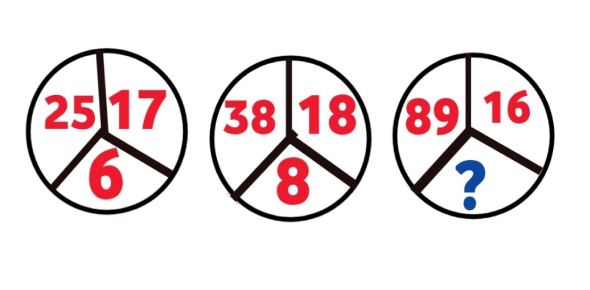SSC CGL Exam Tier-I: Reasoning: Figure Puzzle Quiz!

13 Questions | Total Attempts: 42Settings.

• 1.
Six toys are quite identical to look at, but only one of them is less in weight. It is to be identified using the balance minimum number of times. What is that minimum number of using the balance?
• A.

Once

• B.

2 times

• C.

3 times

• D.

More than 3 times

• 2.
How many triangles are there in the figure PQRS?
• A.

16

• B.

12

• C.

10

• D.

8

• 3.
How many triangles are there in the given figure?
• A.

18

• B.

28

• C.

20

• D.

24

• 4.
How many squares are there in the square figure ABCD?
• A.

16

• B.

17

• C.

26

• D.

30

• 5.
How many rectangles are there in the given figure?
• A.

24

• B.

16

• C.

21

• D.

14

• 6.
Directions: In the following questions, select the related letters/word/number/figure from the given alternatives. How many triangles are there in the following figure?
• A.

16

• B.

13

• C.

9

• D.

7

• 7.
How many rectangles are there in the figure given?
• A.

8

• B.

9

• C.

10

• D.

11

• 8.
How many triangles are there in the following figure?
• A.

11

• B.

13

• C.

9

• D.

15

• 9.
How many triangles are there in the following figure?
• A.

20

• B.

24

• C.

28

• D.

32

• 10.
How many triangles are there in the following figures?
• A.

29

• B.

27

• C.

23

• D.

30

• 11.
How many triangles are there in the given figure?
• A.

16

• B.

8

• C.

14

• D.

12

• 12.
How many rectangles are there in the given diagram?
• A.

4

• B.

7

• C.

9

• D.

18

• 13.
How many triangles are there in the given figure?
• A.

29

• B.

38

• C.

40

• D.

35

Related TopicsBack to top